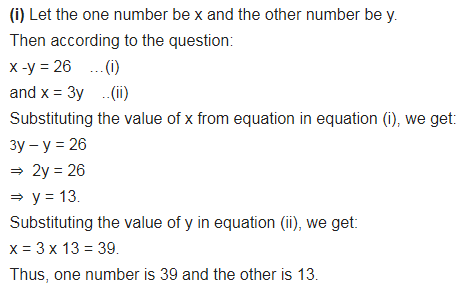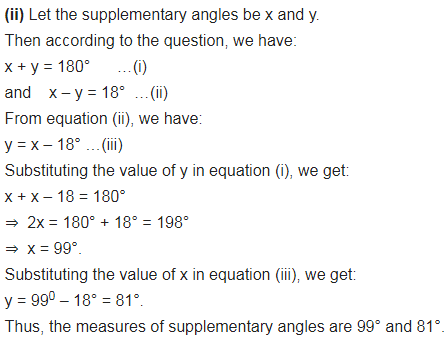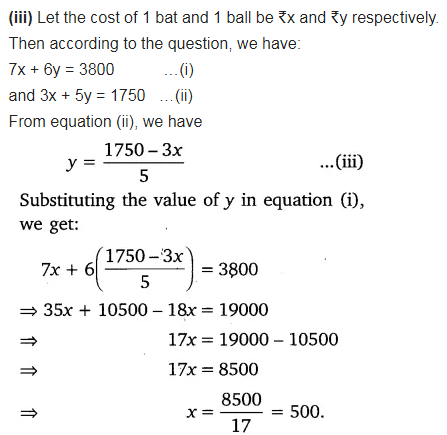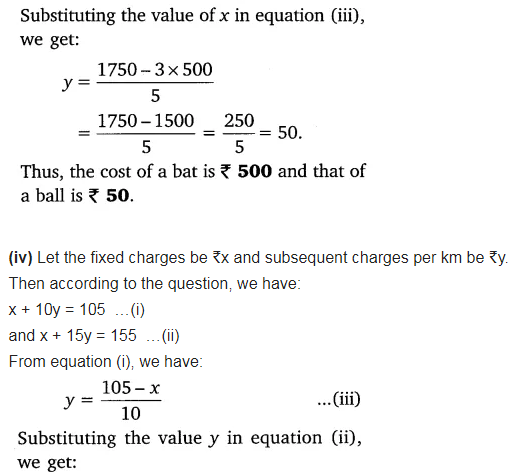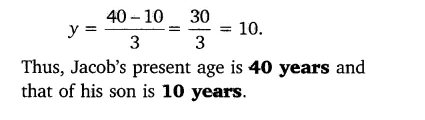# Form the pair of linear equations for the following problems and find their solution

Form the pair of linear equations for the following problems and find their solution by substitution method:
(i) The difference between two numbers is 26 and one number is three times the other. Find them.
(ii) The larger of two supplementary angles exceeds the smaller by 18 degrees. Find them.
(iii) The coach of a cricket team buys 7 bats and 6 balls for ₹3800. Later, she buys 3 bats and 5 balls for ₹1750. Find the cost of each bat and each ball.
(iv) The taxi charges in a city consist of a fixed charge together with the charge for the distance covered. For a distance of 10 km, the charge paid is ₹105 and for a journey of 15 km, the charge paid is ₹155. What are the fixed charges and the charges per km? How much does a person have to pay for travelling a distance of 25 km?
(v) A fraction becomes$\frac { 9 }{ 11 }$, if 2 is added to both the numerator and the denominator. If 3 is added to both the numerator and the denominator, it becomes$\frac { 5 }{ 6 }$. Find the fraction.
(vi) Five years hence, the age of Jacob will be three times that of his son. Five year ago, Jacob’s age was seven times that of his son. What are their present ages?